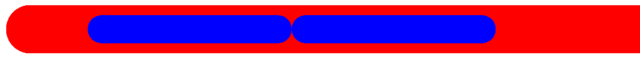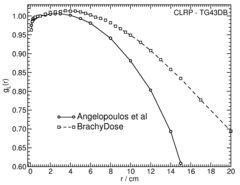# Varian, VariSource VS2000, HDR### Source Description:

Dimensions for the VariSource VS2000 1,2,3 are taken from the study by Angelopoulos et al . The VS2000 consists of two 2.50 mm long sources. Each source is made up of a 2.16 mm long cylindrical section with a 0.34 mm diameter and hemispherical ends with the same diameter. The two sources are contained in a 55.6% / 44.4% - Ni/Ti wire with a diameter of 0.59 mm. The end of the wire was modelled as a hemisphere with its center shifted 3.205 mm from the center of the source. A length of wire extending 5.00 cm from the center of the source was included in this simulation. The active length of this source is 5.00 mm.

### Dose Rate Constant - Λ :

Dose rate constants, Λ , are calculated by dividing the dose to water per history in a (0.1 mm) 3 voxel centered on the reference position, (1 cm,Π/2), in the 80x80x80 cm 3 water phantom, by the air kerma strength per history. Calculations of the air kerma per history are scored in vacuo avoiding the need to correct for attenuation by air. The mass energy absorption coefficients for air used in this calculation are calculated with the composition recommended by TG-43U1 (40% humidity). Air kerma per history is calculated in a 10x10x0.05xcm 3 voxel located 100 cm from the source along the transverse axis and then corrected to give the air kerma per history at a point as described in our previous study 4 . Low energy photons emitted from the source encapsulation are suppressed in the air-kerma calculations by discarding all photons with energy less than 10 keV (i.e. PCUT set to 10 keV in EGSnrc).

### Radial dose function - g(r):

The radial dose function, g(r), is calculated using both line and point source geometry functions and tabulated at 36 different radial distances ranging from 0.2 cm to 20 cm. Fit parameters for a modified polynomial expression are also provided 6 .

Click image for higher res versionTabulated g(r) data: html Excel

### Anisotropy function - F(r,θ):

Anisotropy functions are calculated using the line source approximation and tabulated at 12 radii from 0.25 cm to 20 cm and 47 unique polar angles with a minimum resolution of 5°. The anisotropy factor, φan (r), was calculated by integrating the solid angle weighted dose rate over 0° ≤ ϑ ≤ 180°.

### Along-Away Dose Data:

Along-away dose data are tabulated at 16 away distances from 0 cm to 20 cm and 31 along points from -20 cm to 20 cm. Doses are normalized to s k , the air kerma strength per history.

Tabulated along-away dose data: HTML Excel

### Primary and Scatter Separated (PSS) Dose Data: D ii (r,θ):

Primary and Scatter Separated (PSS) dose data are tabulated at 12 radii from 0.25 cm to 20 cm and 47 unique polar angles with a minimum resolution of 5° . High resolution (Δr = 1 mm, ΔΘ = 1° ) primary scatter dose data are also available in .csv files. For the purposes of these calculations, scatter within the source is not considered and any photon escaping the source encapsulation is considered a primary. Only photons which scatter within the phantom are counted in the scatter tallies. Doses are normalized to the total photon energy escaping the encapsulation.

Click images for higher res versions Tabulated D ii (r,θ) data: Primary , Single Scat , Multiple Scat , Total , All - Excel
High resolution (1mm/1°) Tabulated D ii (r,θ) data in .csv format: Primary , Single Scat , Multiple Scat , Total

### Energy Weighted Photon Spectrum, (dR/dE)/R

The energy weighted photon spectra are scored as:

(dR(E j )/dE)/R = Σ E i / (ΔE·R)

where E j is the energy of the middle of a bin, E i is the energy of the i th photon escaping the encapsulation or cable with energies between Ej ± ΔE/2 and ΔE is the bin width. Energy weighted photon spectra are normalized to the total radiant photon energy, R (total energy of photons escaping the encapsulation/cable) yielding uints of MeV -1 . The spectrum data is available in csv format below. The first line in the csv files contains the number of bins in the file (N), followed by the bin width of all the bins. The next N lines contain the energy of the center of the bin (E j ) followed by the value of (dR(E j )/dE)/R.